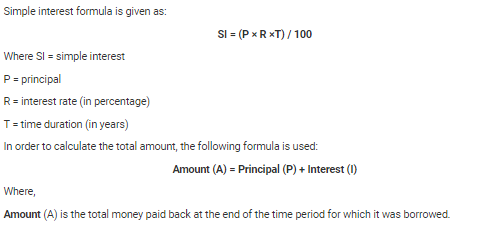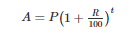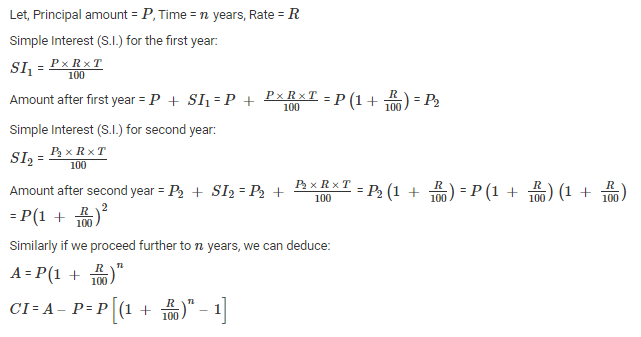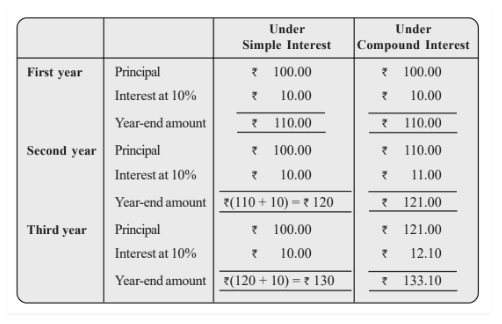# Simple Interest and Compound Interest

Aptitude(Numeracy & Reasoning)- 21st century literacies

Simple Interest and Compound Interest

Lesson Plan

 Objective: To help students understand the two types of interest through application of Simple interest and Compound interest. Students would be able to calculate the interest applicable based on a principal amount given and the rate applicable for a particular amount of time. To enable learners to solve questions of any kind related to S.I & C.I. Students will be able to deduce solutions and develop an ability to understand concepts of interests and its practical applications in daily life. Total Time: 90 Minutes Instructor Note: This unit would deal with the basic nuances of the topic simple interest and compound interest. Trainer is expected to help students learn important variables and factors of this topic such as principal, rates, time etc. Students should be able to understand that money is not free and there is a time value of money. Trainer is expected to equip students with the concepts of interest so well that they are able to use them practically when needed. Owing to the importance of this topic it becomes inevitable to form a strong foundation and holistic learning of the same. Trainer is required to deliver this unit under an engaging environment by using practical examples and competitive activities.  (After explaining concepts & practice) Display/distribute handout consisting of questions. The Foundation section will comprise 4 questions. Instruct students that they will be given 8 minutes to discuss among themselves & solve these 4 questions, whichever group solves maximum problems wins maximum points on the points table (refer opening protocol). Award 5 marks for each correct answer given by a group. Move around the class to check procedures used by students to solve problems. Once time is up, provide solutions and explanations for each question. Switch to moderate level. This section comprises 3 questions and cumulative time allotted will be 10 minutes. All other instructions remain the same. Switch to HOTS. This section comprises 3 questions and cumulative time allotted will be 15 minutes. All other instructions remain the same. Opening Protocol: Greet the participants. Take attendance. Form groups of participants with each group comprising of 4 members.(For entire session) Draw points table on board and ask participants to name their group with some fancy names like “Aptitude thunders”, “Knights of Mathematics”, “Fantastic Statisticians” etc.  Inform the participants that the group which tops the points table will be rewarded at the end of session. Recap the previous session by asking 1-2 participants to share with the class what they learnt and did in the previous session. 3 Minutes Opening Activity: Alright everyone! Before we begin, Tell me when you buy a product, what do you pay the seller? We pay money or anything worth the product.  What do we pay when we buy money or in simple words when we borrow money? When we borrow money, we pay interest to the lender at the rate set by the lender. Money has a time value, for example the value of Rs.10000 in 1980 is not the same in 2021. So it is important for the lender to recover money from the borrower along with interest to keep the time value of money at par. It is very important to understand the concept of interest as we need it in our practical life while borrowing money from a bank, friend, club etc or while lending or investing our money. Before we begin, Let me provide you with a formula of two types of interest and then give you a case study where we will understand the importance of both types of interest and how we may end up losing our money if we are unaware of these concepts. A person named Anshul wanted to buy a car for which he was short of Rs.50000, so he decided to borrow it from the market. He came across two money lenders where one offered him Rs.50000 at 8% simple interest for two years and other offered at 8% compound interest for two years. Anshul, being unaware of two types of interest, is unable to make a decision. Now, this is a situation for him where he can end up taking a loan from any of the lenders as he doesn’t know the difference. How should he take the decision? Help Anshul take his decision backed by your calculation. Groups with right answers will be awarded points on the points table.Trainer’s role- Move around the class while they are solving and give some hints. After students are done with their calculation, provide them with solutions to the above activity and tell them to note the difference.  Alright everyone! Now that you know the important concepts of interest in life and how we might end up our hard earned money if we are unaware of these concepts, Let’s now dive into understanding concepts and subsequently solving problems. 10 Minutes Section 1: Understanding important terms Interest: Interest is payment from a borrower or deposit-taking financial institution to a lender or depositor of an amount above repayment of the principal sum, at a particular rate. Rate of Interest:  An interest rate is the percentage of principal charged by the lender for the use of its money. Principal: Principal refers to the original sum of money borrowed in a loan or put into an investment.  Final Amount: Final amount refers to total amount (Principal amount and Interest) to be paid back to the lender.  Let’s identify these terms in a problem. An amount of Rs.20000 was lend to a borrower for two years at an interest rate of 3% SI. The borrower paid back Rs.21200 to the lender after two years. Here, amount Rs.20000 refers to the principal amount, Two years refers to time by which loan amount along with interest is to be paid back, 3% refers to the percentage of principal charged by the lender and Rs.21200 refers to final amount liable to be paid back to the lender. Section 2: Understanding Simple interest & Compound interest. Simple Interest: Simple interest is the method of calculating the interest amount for some principal amount of money. Simple interest is calculated on the principal, or original, amount of a loan.Compound Interest: Compound interest is the method of calculating the interest amount for some principal amount of money. Compound interest (or compounding interest) is the interest on a loan or deposit calculated based on both the initial principal and the accumulated interest from previous periods. It is different from the simple interest where interest is not added to the principal while calculating the interest during the next period. Compound interest finds its usage in most of the transactions in the banking and finance sectors and also in other areas as well. Some of its applications are: Increase or decrease in population. The growth of bacteria. Rise or depreciation in the value of a product. Compound interest formula is given by: Compound interest = (Amount – Principal) Where the amount is given by,Where, A= Amount, P= Principal, R= Rate of interest and T= Time (in years) Derivation of compound interest formula:Trainer should explain to participants about changes in formula if interest is compounded half yearly and quarterly. Section3: Compound Interest VS Simple Interest: As we know, the major difference between simple interest and compound interest is that in simple interest, interest is calculated on the principal or original sum every year whereas in compound interest, interest is calculated on the principal and also on the interest earned over the years also called interest on interest. Let’s understand the difference between two interests through below example:Section 4: Understanding calculation of SI & CI though percentages: In your previous sessions you have studied the concept of percentages, about multiplying factors etc. now it’s time to utilise those concepts in solving SI and CI problems. Let us see some examples. Example 1: What is SI of Rs.800 on 5% per annum for 3 years? We can either put formula and calculate as below: SI= (800*5*3)/100 = 120 Or, As we know that in simple interest, interest is always calculated on principal so we can just calculate 15% (5% for 3 years) of 800 which is 120. Thus, in this way we don’t have to remember the formula and we can simply get answers by using the concept of percentages. Example 2: What will be the CI obtained on an amount of Rs.4800 at the rate of 5% for 3 years? We can either put formula and calculate as below: Amount= 4800[1+ (5/100)]^3 = 5556.6 ; CI= (5556.6 – 4800)= 756.6 Or, We can simply use concept of multiplying factor learnt in percentages, since 4800 is increasing by 5% every year for constant 3 years, we can write it as  4800* (1.05)^3 = 5556.6 ; CI = (5556.6-4800) = 756.6. Thus, in this way we don’t have to remember the complex formula of CI and we easily solve problems based on CI through the concept of multiplying factor learnt in session of percentage. Let’s solve a few more questions and the groups will be rewarded points based on their answers. 1. A sum of Rs.15000 amount gave Rs.4500 as interest in 5 years. What is the rate of interest? Answer- 6% 2. What would be the C.I on Rs.17500 at the rate of 12p.c.pa after 2 years? Answer- 4452 3. What would be C.I obtained on an amount of 12500 at the rate of 12p.c.pa after 2 years? Answer- 3180 4. Find the CI on Rs.12500 at 8% per annum for 9 months compounded quarterly. Answer- 765 Trainer should recap the session of percentages and repeat concepts of multiplying factors taught and help students solve problems through those concepts. 30 Minutes